# 新特性

comeweijjd枫长LH...大约 13 分钟

### # 1. 请简述C++11中的右值引用和移动语义。

C++11中的右值引用和移动语义是为了解决性能问题和提高资源利用率而引入的新特性。

``````class MyClass {
public:
MyClass(MyClass &&other) {
// 移动构造函数实现
}
};
``````

``````class MyClass {
public:
MyClass& operator=(MyClass &&other) {
// 移动赋值运算符实现
return *this;
}
};
``````

### # 2. 在C++11及以后版本中，如何使用lambda表达式？

``````[capture](parameters) -> return_type { body }
``````

• capture：捕获子句，用于指定lambda表达式需要访问的外部变量。可以捕获变量值（使用值捕获）或捕获变量引用（使用引用捕获）。
• parameters：参数列表，与普通函数的参数列表类似。如果没有参数，可以省略括号。
• return_type：返回类型，可选。如果没有指定返回类型，编译器会根据函数体中的return语句自动推导。
• body：函数体，包含lambda表达式的逻辑代码。

``````// 无参数和返回值的lambda表达式
auto print_hello = []() { std::cout << "Hello, world!" << std::endl; };
print_hello(); // 输出 "Hello, world!"

// 带参数的lambda表达式
auto add = [](int a, int b) { return a + b; };
std::cout << add(3, 5) << std::endl; // 输出 8

// 带捕获的lambda表达式
int x = 10;
int y = 20;
auto add_with_capture = [x, &y](int a, int b) { return a + b + x + y; };
std::cout << add_with_capture(3, 5) << std::endl; // 输出 38

// 带返回类型的lambda表达式
auto multiply = [](double a, double b) -> double { return a * b; };
std::cout << multiply(3.5, 2.0) << std::endl; // 输出 7.0
``````

lambda表达式在STL算法中使用，如`std::sort``std::find_if`等，或在异步编程、回调函数等场景中很有用。

### # 3. C++14中的泛型Lambda是什么？请举例说明。

C++14中的泛型lambda指的是可以接受泛型参数的lambda表达式。这种lambda表达式可以处理不同类型的参数，而无需为每种类型编写单独的lambda表达式。泛型lambda的参数列表中的参数类型使用关键字`auto`指定，编译器会根据实际传入的参数类型自动推导出对应的类型。

``````// 泛型lambda，可以处理不同类型的参数
auto add = [](auto a, auto b) { return a + b; };

std::cout << add(3, 5) << std::endl;         // 输出 8 (整数相加)
std::cout << add(3.5, 2.0) << std::endl;     // 输出 5.5 (浮点数相加)
std::cout << add(std::string("Hello, "), "world!") << std::endl; // 输出 "Hello, world!" (字符串拼接)
``````

``````std::vector<int> int_vec = {3, 1, 4, 1, 5, 9};
std::vector<double> double_vec = {3.1, 1.4, 1.5, 9.2};

// 使用泛型lambda进行排序
auto greater_than = [](auto a, auto b) { return a > b; };

std::sort(int_vec.begin(), int_vec.end(), greater_than);
std::sort(double_vec.begin(), double_vec.end(), greater_than);

// int_vec: {9, 5, 4, 3, 1, 1}
// double_vec: {9.2, 3.1, 1.5, 1.4}
``````

### # 4. 请解释C++17中的结构化绑定（structured bindings）及其用法。

``````#include <iostream>
#include <map>
#include <string>

int main() {
std::map<std::string, int> my_map = {{"Alice", 30}, {"Bob", 25}, {"Eve", 22}};

for (const auto& [name, age] : my_map) {
std::cout << name << " is " << age << " years old." << std::endl;
}

return 0;
}
``````

``````#include <iostream>
#include <tuple>

int main() {
std::tuple<int, std::string, float> my_tuple(42, "hello", 3.14f);

auto [num, str, flt] = my_tuple;
std::cout << "num: " << num << ", str: " << str << ", flt: " << flt << std::endl;

return 0;
}
``````

### # 5. 在C++17中，如何使用if constexpr进行编译时条件编译？

`if constexpr`是一种条件编译特性，允许在编译时根据常量表达式的值选择执行不同的代码分支。对于泛型编程和模板元编程更有用，因为可以帮助减少模板特化的数量，并简化代码。使用`if constexpr`可以避免不必要的模板特化，并提高编译效率。

`if constexpr`的语法如下：

``````if constexpr (condition) {
// 代码块1：当condition为true时执行
} else {
// 代码块2：当condition为false时执行
}
``````

`condition`是一个常量表达式，它在编译时必须是可计算的。`if constexpr`会根据`condition`的值，仅保留一个代码分支，丢弃另一个。未选择的代码分支在编译时会被完全忽略，不会生成任何目标代码。

``````#include <iostream>
#include <type_traits>

template <typename T>
void print(const T& value) {
if constexpr (std::is_integral_v<T>) {
std::cout << "Integral value: " << value << std::endl;
} else if constexpr (std::is_floating_point_v<T>) {
std::cout << "Floating-point value: " << value << std::endl;
} else {
std::cout << "Other value: " << value << std::endl;
}
}

int main() {
int i = 42;
double d = 3.14;
std::string s = "hello";

print(i); // 输出：Integral value: 42
print(d); // 输出：Floating-point value: 3.14
print(s); // 输出：Other value: hello

return 0;
}
``````

`print`函数根据参数`value`的类型选择性地输出不同的信息。使用`if constexpr`语句和`std::is_integral_v``std::is_floating_point_v`这些类型特性来检查参数类型。编译器会根据`T`的类型，在编译时选择执行相应的代码分支。

### # 6. C++20中的概念（concepts）是什么？请举例说明。

``````#include <iostream>
#include <concepts>

// 定义一个概念，要求类型T支持`<`运算符
template<typename T>
concept LessThanComparable = requires(T a, T b) {
{ a < b } -> std::convertible_to<bool>;
};

// 使用概念约束模板参数类型，要求类型T满足LessThanComparable概念
template<LessThanComparable T>
T minimum(T a, T b) {
return a < b ? a : b;
}

int main() {
std::cout << minimum(3, 5) << std::endl;         // 输出 3
std::cout << minimum(3.5, 2.0) << std::endl;     // 输出 2.0
std::cout << minimum(std::string("abc"), "xyz") << std::endl; // 输出 "abc"
}
``````

C++20的标准库还引入了许多内置的概念，例如`std::equality_comparable``std::copyable``std::integral`等。

### # 7. 请解释C++20中的三大运算符：<=>（三路比较运算符），&&=（逻辑与复合赋值运算符），||=（逻辑或复合赋值运算符）。

`<=>`（三路比较运算符）

“三路比较运算符”（Spaceship Operator）也被称为“默认比较运算符”。它用于同时比较两个对象的大小，返回一个`std::strong_ordering``std::weak_ordering``std::partial_ordering``std::strong_equality`类型的值。这个运算符可以简化自定义类型的比较，并减少比较运算符（如`<``<=``>``>=``==``!=`）的重载数量。

``````#include <compare>
#include <iostream>

struct Point {
int x, y;

auto operator<=>(const Point& other) const = default;
};

int main() {
Point p1 = {3, 4};
Point p2 = {3, 5};

if (p1 < p2) {
std::cout << "p1 is less than p2" << std::endl;
} else {
std::cout << "p1 is not less than p2" << std::endl;
}

return 0;
}
``````

`Point`结构体定义了一个默认的三路比较运算符，就可以使用标准的比较运算符（如`<``<=`等）来比较`Point`对象。

`&&=`（逻辑与复合赋值运算符）

``````bool a = true;
bool b = false;

a &&= b; // 等价于 a = a && b，a的值将变为false
``````

`||=`（逻辑或复合赋值运算符）

``````bool a = true;
bool b = false;

a ||= b; // 等价于 a = a || b，a的值保持不变（true）
``````

### # 8. 请简述C++20中的协程（coroutines）及其用途。

C++20中的协程（coroutines）是一种新的编程范式，用于简化异步编程和非阻塞代码的编写。协程是一种可以被暂停和恢复执行的特殊函数。当一个协程暂停时，它会保存当前的执行状态（包括局部变量和执行位置），以便在之后的某个时刻恢复执行。协程可以让我们编写更简洁、更易读的异步代码，避免回调地狱（callback hell）和复杂的状态管理。

C++20中的协程主要涉及以下几个关键字和概念：

• `co_await`：在协程中，可以使用`co_await`表达式暂停协程的执行，等待某个异步操作完成。当异步操作完成后，协程会在`co_await`表达式之后的位置恢复执行。
• `co_return``co_return`用于从协程中返回一个值。它类似于普通函数中的`return`语句，但适用于协程。
• `co_yield``co_yield`用于在协程中产生一个值并暂停执行。当协程被恢复时，它将从`co_yield`表达式之后的位置继续执行。
• `std::coroutine_handle``std::coroutine_handle`是一个模板类，用于表示和操作协程。它提供了一些操作，如`resume()`（恢复协程执行）和`done()`（检查协程是否已完成）等。
• `std::suspend_always``std::suspend_never`：这两个类分别表示协程在某个点上始终暂停和永不暂停。它们通常用于定义协程的“promise”对象，以控制协程的暂停和恢复行为。

``````#include <iostream>
#include <coroutine>
#include <future>

// 异步操作，返回一个std::future<int>
std::future<int> async_operation() {
return std::async([]() { return 42; });
}

// 协程函数，使用co_await等待异步操作完成
std::future<void> example_coroutine() {
int result = co_await async_operation(); // 使用co_await等待异步操作完成
std::cout << "Result: " << result << std::endl;
}

int main() {
std::future<void> fut = example_coroutine(); // 调用协程
fut.wait(); // 等待协程执行完成
}
``````

``````template<typename T>
struct future_awaiter {
std::future<T> f;

}

void await_suspend(std::coroutine_handle<> h) {
f.wait();
h.resume();
}).detach();
}

T await_resume() {
return f.get();
}
};

template<typename T>
future_awaiter<T> operator co_await(std::future<T> &&f) {
return { std::move(f) };
}
``````

### # 9: 请介绍C++20中的范围for循环（range-based for loop）的新用法。

range-based for loop支持初始化语句（init-statement）。这使得在进入循环之前，可以在范围for循环的语法中声明和初始化一个或多个变量。在需要在循环范围内共享一个状态或资源时非常有用。

``````for (init-statement; range_declaration : range_expression) {
// 循环体
}
``````

`init-statement`是一个可选的初始化语句，它在循环开始之前执行。这个初始化语句的作用域在整个循环范围内，包括循环体和`range_expression`

``````#include <iostream>
#include <vector>

int main() {
std::vector<int> numbers = {1, 2, 3, 4, 5};

for (int sum = 0; const auto& num : numbers) {
sum += num;
std::cout << "Current sum: " << sum << std::endl;
}

return 0;
}
``````

### # 10 . C++20中的模块（modules）是什么？请简要介绍其优点和用法。

• 提高编译速度：模块可以显著降低编译时间，因为它们只需要编译一次，然后在其他地方导入时直接使用编译好的结果，而无需像传统头文件那样每次都重新解析和编译。
• 减少命名空间污染：模块中的符号默认是不可见的，只有通过导出（export）显式声明的符号才能在其他地方导入。这减少了不同库和代码之间的命名冲突风险。
• 更好的封装和隔离：模块提供了更强的封装，因为它们允许开发者仅导出需要公开的接口，而隐藏实现细节。

``````// my_module.cppm
export module my_module;

export int add(int a, int b) {
return a + b;
}

int hidden_function(int a, int b) {
return a * b;
}
``````

``````// main.cpp
import my_module;

#include <iostream>

int main() {
std::cout << "The sum is: " << result << std::endl;
return 0;
}
``````

`main.cpp`文件中，导入`my_module`模块并调用了`add`函数。

•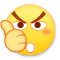0
•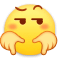0
•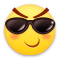0
•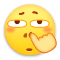0
•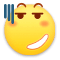0
•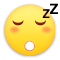0

• 按正序
• 按倒序
• 按热度